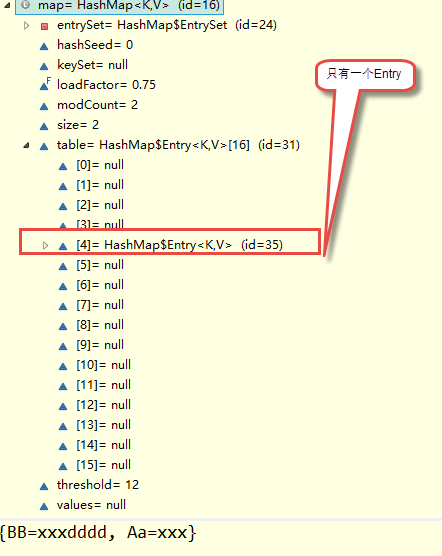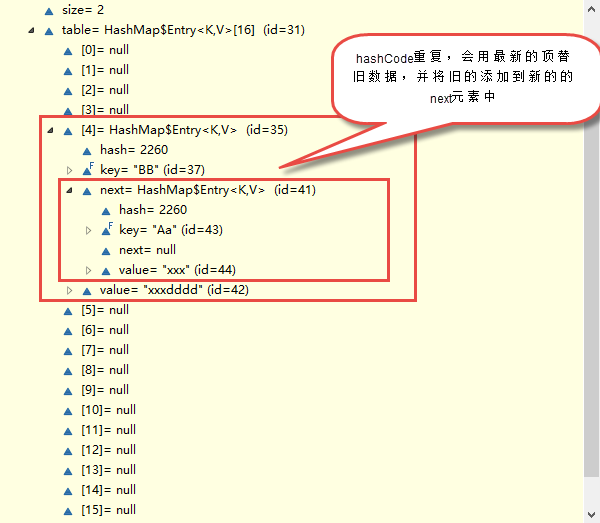# 一次性搞清楚equals和hashCode

2018/03/06 17:55

在程序设计中，有很多的“公约”，遵守约定去实现你的代码，会让你避开很多坑，这些公约是前人总结出来的设计规范。

Object类是Java中的万类之祖，其中，equals和hashCode是2个非常重要的方法。

这2个方法总是被人放在一起讨论。最近在看集合框架，为了打基础，就决定把一些细枝末节清理掉。一次性搞清楚！

## public boolean equals(Object obj)

Object类中默认的实现方式是  :   return this == obj  。那就是说，只有this 和 obj引用同一个对象，才会返回true。(如果我们没有重写equals方法，equals和==一样比较的都是内存地址，只是equals可以让我们重写。)

而我们往往需要用equals来判断 2个对象是否等价，而非验证他们的唯一性。这样我们在实现自己的类时，就要重写equals.

一个例子

class Test
{
private int num;
private String data;

public boolean equals(Object obj)
{
if (this == obj)
return true;

if ((obj == null) || (obj.getClass() != this.getClass()))
return false;

//能执行到这里，说明obj和this同类且非null。
Test test = (Test) obj;
return num == test.num&& (data == test.data || (data != null && data.equals(test.data)));
}

public int hashCode()
{
//重写equals，也必须重写hashCode。具体后面介绍。
}

}

## equals编写指导

Test类对象有2个字段，num和data，这2个字段代表了对象的状态，他们也用在equals方法中作为评判的依据。

1、有些程序员使用下面的第二种写法替代第一种比较运行时类的写法。应该避免这样做。

if((obj == null) || (obj.getClass() != this.getClass()))
return false;
if(!(obj instanceof Test))
return false; // avoid 避免！

dog instanceof Animal      得到true

animal instanceof Dog      得到false

animal.equls(dog) 返回true
dog.equals(animal) 返回false

2、按照第一种方法实现，那么equals只能比较同一个类的对象，不同类对象永远是false。但这并不是强制要求的。一般我们也很少需要在不同的类之间使用equals。

3、在具体比较对象的字段的时候，对于基本值类型的字段，直接用 == 来比较（注意浮点数的比较，这是一个坑）对于引用类型的字段，你可以调用他们的equals，当然，你也需要处理字段为null 的情况。对于浮点数的比较，我在看Arrays.binarySearch的源代码时，发现了如下对于浮点数的比较的技巧：

if ( Double.doubleToLongBits(d1) == Double.doubleToLongBits(d2) ) //d1 和 d2 是double类型

if(  Float.floatToIntBits(f1) == Float.floatToIntBits(f2)  )      //f1 和 f2 是d2是float类型

4、并不总是要将对象的所有字段来作为equals 的评判依据，那取决于你的业务要求。比如你要做一个家电功率统计系统，如果2个家电的功率一样，那就有足够的依据认为这2个家电对象等价了，至少在你这个业务逻辑背景下是等价的，并不关心他们的价钱啊，品牌啊，大小等其他参数。

5、最后需要注意的是，equals 方法的参数类型是Object，不要写错！

## public int hashCode()

这个方法返回对象的散列码，返回值是int类型的散列码。
对象的散列码是为了更好的支持基于哈希机制的Java集合类，例如 Hashtable, HashMap, HashSet 等。

合乎情理的是：同一个类中的不同对象返回不同的散列码。典型的方式就是根据对象的地址来转换为此对象的散列码，但是这种方式对于Java来说并不是唯一要求的的实现方式。通常也不是最好的实现方式。
相比 于 equals公认实现约定，hashCode的公约要求是很容易理解的。有2个重点是hashCode方法必须遵守的。约定的第3点，其实就是第2点的化，下面我们就来看看对hashCode方法的一致约定要求。

## hashCode编写指导

1、定义一个int类型的变量 hash,初始化为 7。

接下来让你认为重要的字段（equals中衡量相等的字段）参入散列运，算每一个重要字段都会产生一个hash分量，为最终的hash值做出贡献（影响）

byte, char, short ， int (int)var
long  (int)(var ^ (var >>> 32))
boolean var?1:0
float  Float.floatToIntBits(var)
double  long bits = Double.doubleToLongBits(var);

引用类型   (null == var ? 0 : var.hashCode())

int hash = 7;

hash = 31 * hash + 字段1贡献分量;

hash = 31 * hash + 字段2贡献分量;

.....

return hash；

== 和  equals()都是比较内存地址，只是==不能重写，而equals可以重写

hashcode返回一个int型的hash地址。

package CollectionTest;

public class Person {

private String name;
private int age;
public String getName() {
return name;
}
public void setName(String name) {
this.name = name;
}
public int getAge() {
return age;
}
public void setAge(int age) {
this.age = age;
}
@Override
public int hashCode() {
// TODO Auto-generated method stub
return this.name.hashCode()+age;
}
@Override
public boolean equals(Object obj) {
if (!(obj instanceof Person)) {
return false;
}
Person p = (Person) obj;
return this.name.equals(p.name) && this.age == p.age;
}
protected Person(String name, int age) {
super();
this.name = name;
this.age = age;
}

}

public static void main(String[] args) {
Person p1 = new Person("qz", 25);
Person p2 = new Person("qz", 25);
System.out.println(p1.equals(p2));//true
System.out.println(p1==p2);//false
System.out.println(p1.toString());//CollectionTest.Person@e42
}

### 1.Java中String的hashCode()与equals()源码查看:

String实现原理(基于char[]实现):不可变类，每次值都是存放在char[]数组中，并且进行深复制。

public final class String
implements java.io.Serializable, Comparable<String>, CharSequence {

private final char value[];

private int hash; // Default to 0

,,,
}

hashCode()源码查看:

public int hashCode() {
int h = hash;//默认为0
if (h == 0 && value.length > 0) {
char val[] = value;

for (int i = 0; i < value.length; i++) {
h = 31 * h + val[i];
}
hash = h;
}
return h;
}

String s1 = "a";
String s2 = "b";
System.out.println(s1.hashCode());//97
System.out.println(s2.hashCode());//98

equals(obj)源码查看:　　是将形参转变为String，然后遍历里面的char[]，两个char[]进行依次对比。也就是比较字符串的值是否相等。

public boolean equals(Object anObject) {
if (this == anObject) {
return true;
}
if (anObject instanceof String) {
String anotherString = (String) anObject;
int n = value.length;
if (n == anotherString.value.length) {
char v1[] = value;
char v2[] = anotherString.value;
int i = 0;
while (n-- != 0) {
if (v1[i] != v2[i])
return false;
i++;
}
return true;
}
}
return false;
}

package cn.qlq.test;

import java.util.HashMap;

public class ArrayTest {
public static void main(String[] args) {
String s1 = "Aa";
String s2 = "BB";
System.out.println(s1.hashCode());
System.out.println(s2.hashCode());

HashMap map = new HashMap();
map.put(s1, "xxx");
map.put(s2, "xxxdddd");
System.out.println(map);
}

}

2112
2112
{BB=xxxdddd, Aa=xxx}

"Aa" 与"BB"的hashCode相等，那么是如何存入map的?--验证hashmap的实现原理基于数组+链表先存入Aa，并放在第五个数组位置，当存BB的时候发现hashCode一样，会将BB存到第五个位置，并将第五个位置元素的next(也是一个Entry)存为Aa。也就是数组加链表实现原理。

### 2.8种基本数据类型的包装类型研究，以Integer为例

先看一段代码:

package cn.qlq.test;

public class ArrayTest {
public static void main(String[] args) {
Integer i1 = new Integer(1);
Integer i2 = new Integer(1);
System.out.println(i1.hashCode());
System.out.println(i2.hashCode());
System.out.println(i1 == i2);
System.out.println(i1.equals(i2));

System.out.println("-------------------");

Integer i3 = 1;
Integer i4 = 1;
System.out.println(i3.hashCode());
System.out.println(i4.hashCode());
System.out.println(i3 == i4);
System.out.println(i3.equals(i4));

}

}

1
1
false
true
-------------------
1
1
true
true

Integer i3=1；的时候是从常量池中查找值为1的常量，i3指向该常量;Integer i4=1的时候会直接指向该常量，所以 i3 == i4为true。注意integer的常量池只有-128到127.测试如下:

package cn.qlq.test;

public class ArrayTest {
public static void main(String[] args) {

Integer i3 = -128;
Integer i4 = -128;
Integer i33 = 128;
Integer i44 = 128;
System.out.println(i3 == i4);
System.out.println(i33 == i44);

}

}

true
false

1.Integer的hashCode()实现原理---直接返回的是值

public int hashCode() {
return value;
}

value是其内部的一个int属性:(---也是其不可变类的实现原理)

public static Integer valueOf(String s, int radix) throws NumberFormatException {
}

public static Integer valueOf(String s) throws NumberFormatException {
return Integer.valueOf(parseInt(s, 10));
}

private static class IntegerCache {
static final int low = -128;
static final int high;
static final Integer cache[];

static {
// high value may be configured by property
int h = 127;
String integerCacheHighPropValue =
sun.misc.VM.getSavedProperty("java.lang.Integer.IntegerCache.high");
if (integerCacheHighPropValue != null) {
int i = parseInt(integerCacheHighPropValue);
i = Math.max(i, 127);
// Maximum array size is Integer.MAX_VALUE
h = Math.min(i, Integer.MAX_VALUE - (-low) -1);
}
high = h;

cache = new Integer[(high - low) + 1];
int j = low;
for(int k = 0; k < cache.length; k++)
cache[k] = new Integer(j++);
}

private IntegerCache() {}
}

public static Integer valueOf(int i) {
assert IntegerCache.high >= 127;
if (i >= IntegerCache.low && i <= IntegerCache.high)
return IntegerCache.cache[i + (-IntegerCache.low)];
return new Integer(i);
}

private final int value;

public Integer(int value) {
this.value = value;
}

2.Integer的equals(obj)源码查看:  也是直接 比较两者的内部的value值

public boolean equals(Object obj) {
if (obj instanceof Integer) {
return value == ((Integer)obj).intValue();
}
return false;
}

也就是8种基本数据类型的包装类型和包装类型比较值的时候用equals比较，比较内存的时候用  ==

其他我们的引用类型一般equals是比较内存地址，我们可以重写equals()方法进行比较。0 评论
0 收藏
0